• 您可以将集合编码为整数：整数的第i位表示第i个城市的状态(即，我们是否将其放入子集中)。例如，3510=1000112将表示城市{1，2，6}。这里我从最右边的一位开始计数，代表城市1。在为了使用子集的这种表示来索引列表，...
您可以将集合编码为整数：整数的第i位表示第i个城市的状态(即，我们是否将其放入子集中)。例如，3510=1000112将表示城市{1，2，6}。这里我从最右边的一位开始计数，代表城市1。在为了使用子集的这种表示来索引列表，您应该创建长度为2n的二维数组：# Assuming n is given.A = [[0 for i in xrange(n)] for j in xrange(2 ** n)]这是因为使用n位整数可以表示{1，2，…，n}的每一个子集(请记住，每一位正好对应于一个城市)。在此表示为您提供了许多不错的可能性：^{pr2}\$这是我如何实现您的伪代码(警告：没有进行测试)：# INFINITY and n are defined somewhere above.A = [[INFINITY for i in xrange(n)] for j in xrange(2 ** n)]# Base case (I guess it should read "if S = {1}, then A[S, 1] = 0",because otherwise S = {0} is not a valid index to A, according to line #1)A = 0# Iterate over all subsets:subsets = range(1, 2 ** n)for subset in sorted(subsets, key=lambda x: bin(x).count('1')):if not subset & 1:# City #1 is not presented.continuefor j in xrange(2, n + 1):if not (1 << (j - 1)) & subset:# City #j is not presented.continuefor k in xrange(1, n + 1):if k == j or not (1 << (k - 1)) & subset:continueA[subset][j] = min(A[subset][j], A[subset ^ (1 << (j - 1))][k] + get_dist(j, k))除了拥有实现伪代码所需的所有功能外，这种方法比tuples\dicts更快。在
展开全文• 本介绍用python解决TSP问题的第二个方法——动态规划法算法介绍动态规划算法根据的原理是，可以将原问题细分为规模更小的子问题，并且原问题的最优解中包含了子问题的最优解。也就是说，动态规划是一种将问题实例...
本介绍用python解决TSP问题的第二个方法——动态规划法算法介绍动态规划算法根据的原理是，可以将原问题细分为规模更小的子问题，并且原问题的最优解中包含了子问题的最优解。也就是说，动态规划是一种将问题实例分解为更小的、相似的子问题，并存储子问题的解而避免计算重复的子问题，以解决最优化问题的算法策略。我使用DP求解TSP问题的主要分为三个主要部分：1)     假定我们从城市0出发，经过了所有城市，并返回到城市0。那么我们需要记录的信息有：当前所在城市location，当前未遍历的城市集合s。2)     状态转移方程，状态转移方程是DP算法的核心部分，它代表了子问题和原问题的关系，通过状态转移方程可以将原问题不断细分为各个子问题。我们状态转移方程的定义如下所示：T(s,init)代表的意思是从init点出发经过s中全部的点回到init的距离。3)     构建T表记录T的值，如果不去记录每次递归的T值，那么以后每次搜索都要重新计算，就成了暴力搜索。所以我们构建一个T表dp[s][init]，记录每次求出来的T函数值，即将T(s,init)的值记录在dp[s][init]位置。程序输入：1 2066 23332 935 13043 1270 2004 1389 7005 984 28106 2253 4787 949 30258 87 24839 3094 188310 2706 3130代码："""动态规划法name:xxxdate:6.8"""import pandas as pdimport numpy as npimport mathimport timedataframe = pd.read_csv("./data/TSP10cities.tsp",sep=" ",header=None)v = dataframe.iloc[:,1:3]train_v= np.array(v)train_d=train_vdist = np.zeros((train_v.shape,train_d.shape))#计算距离矩阵for i in range(train_v.shape):for j in range(train_d.shape):dist[i,j] = math.sqrt(np.sum((train_v[i,:]-train_d[j,:])**2))"""N:城市数s:二进制表示，遍历过得城市对应位为1，未遍历为0dp:动态规划的距离数组dist：城市间距离矩阵sumpath:目前的最小路径总长度Dtemp：当前最小距离path:记录下一个应该到达的城市"""N=train_v.shapepath = np.ones((2**(N+1),N))dp = np.ones((2**(train_v.shape+1),train_d.shape))*-1def TSP(s,init,num):if dp[s][init] !=-1 :return dp[s][init]if s==(1<return dist[init]sumpath=1000000000for i in range(N):if s&(1<m=TSP(s&(~(1<if msumpath=mpath[s][init]=idp[s][init]=sumpathreturn dp[s][init]if __name__ == "__main__":init_point=0s=0for i in range(1,N+1):s=s|(1<start = time.clock()distance=TSP(s,init_point,0)end = time.clock()s=0b11111111110init=0num=0print(distance)while True:print(path[s][init])init=int(path[s][init])s=s&(~(1<num+=1if num>9:breakprint("程序的运行时间是：%s"%(end-start))结果：展开全文• } int TSP(){ for (int i = 1; i ; ++i) {//初始化第0列,i为行数 distanceTable[i] = distanceMatrix[i]; } //第一步，先不填最后一个空，先填前面的 for (int j = 1; j (int)pow(2,N - 1); ++j) {//从第一列...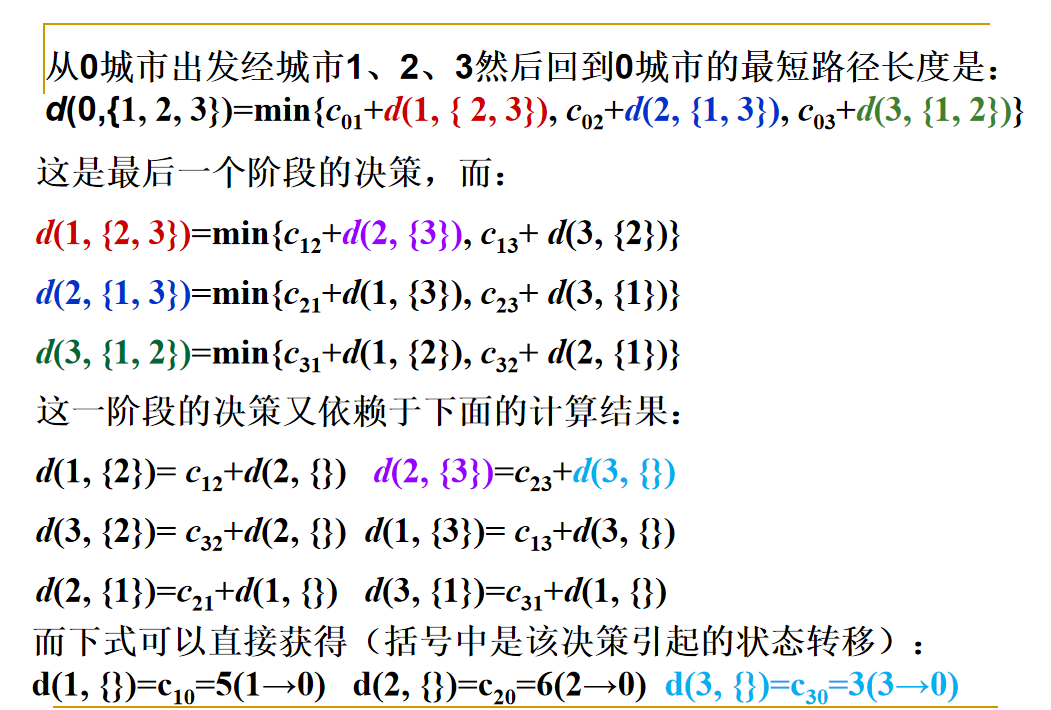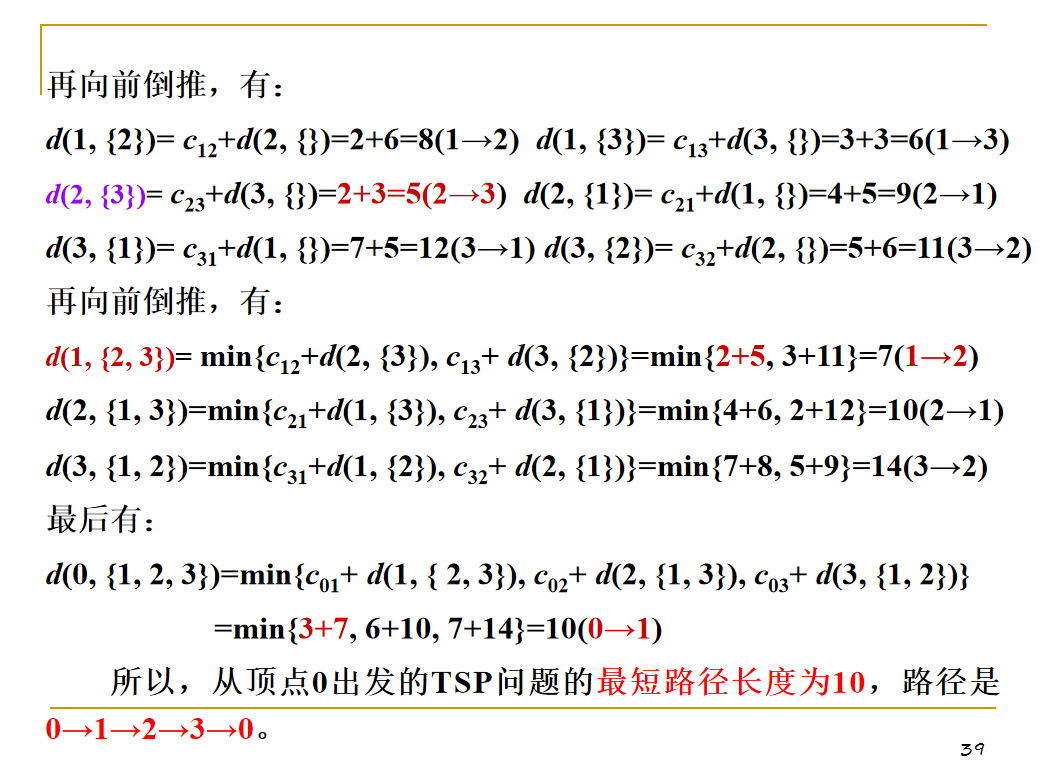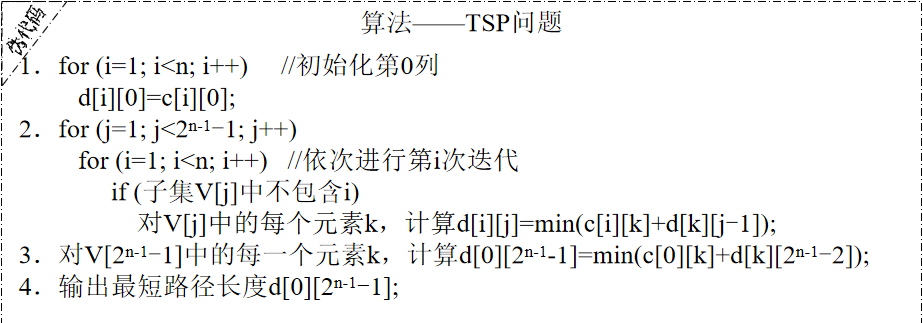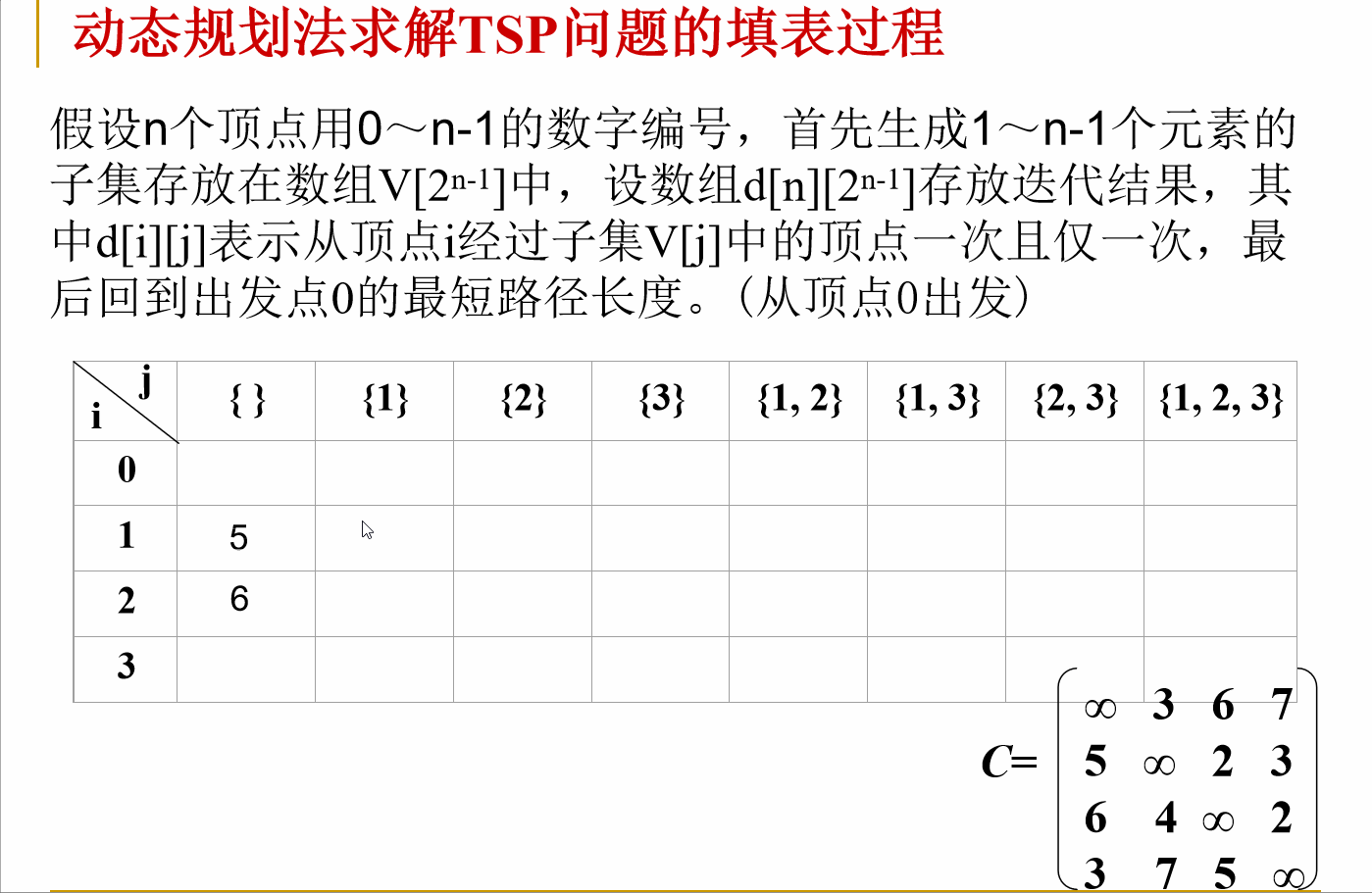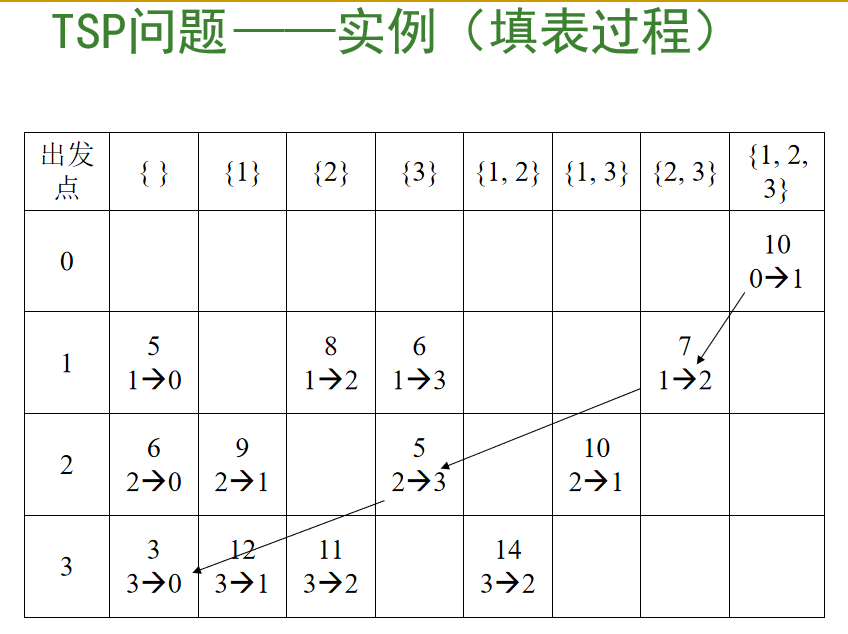#include <iostream>
#include <cmath>
using namespace std;
//集合虚拟化用000 、001 、010 、011 、100 、101 、110 、111分别表示{} 、{1}（V[2^(1-1)]） 、{2}（V[2^(2-1)]） 、{1,2}（V[2^(1-1)+2^(2-1)]） 、{3}（V[2^(3-1)]） 、{1,3}（V[2^(1-1)+2^(3-1)]） 、{2,3}（V[2^(2-1)+2^(3-1)]） 、{1,2,3}（V[2^(1-1)+2^(2-1)+2^(3-1)]）注意{1,2} 、{3}顺序
//这是本题的难点，将十进制数转化为二进制
//①直观的看出来包含的元素②可以直接用编码表示这个集合
int distanceTable;//需要填的表格
int distanceMatrix;//城市距离的代价矩阵
int N;//城市数量
int path;//若path[i][j] = k,则说明从i到集合V[j]的最短距离是从i到集合V[j]中的k
int cinDistance(){//输入代价矩阵
cout<<"请输入城市个数"<<endl;
cin>>N;
cout<<"请输入城市距离的代价矩阵"<<endl;
for (int i = 0; i < N; ++i) {
for (int j = 0; j < N; ++j) {
cin>>distanceMatrix[i][j];
}
}
for (int k = 0; k < N; ++k) {//规范
distanceMatrix[k][k] = 0;
}
cout<<"您的代价矩阵已被规范化"<<endl;
for (int i = 0; i < N; ++i) {
for (int j = 0; j < (int)pow(2,N); ++j) {
distanceTable[i][j] = -1;
}
}
return 0;
}

int exist(int i,int j){//判断集合V[j]中是否有元素i,有则返回1
if((int)pow(2,i - 1)&j)return 1;
else return 0;
}

int TSP(){
for (int i = 1; i < N; ++i) {//初始化第0列,i为行数
distanceTable[i] = distanceMatrix[i];
}
//第一步，先不填最后一个空，先填前面的
for (int j = 1; j < (int)pow(2,N - 1); ++j) {//从第一列开始填表,j为列数
for (int i = 1; i < N; ++i) {//从第一行开始
int min = 10000;
if(!exist(i,j)){//如果集合V[j]中没有元素i，则求i到V[j]再到0的最短路径
for (int k = 1; k < N; ++k) {
if(exist(k,j)){//如果k存在集合V[j]中，则求i到k + (k再到0的最短距离)
//
int d = distanceMatrix[i][k] + distanceTable[k][j - (int)pow(2,k - 1)];//这一行很难理解，为什么V[j]中除掉看，就是
//集合V[j - (int)pow(2,k - 1)]呢，因为 例如V={1,3}他的编码是101，如果去掉3，即是101 - 2的3次方 = 101 - 100 = 001，可知集合只剩下1了,所以就是集合001了V
/
if(d < min){
path[i][j] = k;//即从i到V[j]再到0的最短距离是先从i到k
min = d;
}
}
}
distanceTable[i][j] = min;
}
}
}
//填最后一个空也就是求最短路径长度，并求最短路径
int min = 1000;
for (int k = 1; k < N; ++k) {
int d = distanceMatrix[k] + distanceTable[k][((int)pow(2,N - 1) - 1) - (int)pow(2,k - 1)];
if(d < min){
min = d;
path[(int)pow(2,N - 1) - 1] = k;
}
}
distanceTable[((int)pow(2,N - 1) - 1)] = min;
return 0;
}

int coutResult(){
for (int i = 0; i < N; ++i) {//输出表格
for (int j = 0; j < (int)pow(2,N - 1); ++j) {
cout<<distanceTable[i][j]<<'\t';
}
cout<<endl;
}
//输出路径
cout<<"0->";
int i = 0;
for (int j = (int)pow(2,N - 1) - 1; j > 0;) {
i = path[i][j];
cout<<i<<"->";
j = j - (int)pow(2,i - 1);
}
cout<<"0"<<endl;
return 0;
}

int main()
{
cinDistance();
TSP();
coutResult();
return 0;
}




展开全文• 动态规划问题使用GA和PSO算法求解10个城市TSP问题动态规划
• 旅行商问题，即TSP问题（Traveling Salesman Problem）又译为旅行推销员问题、货郎担问题，是数学领域中著名问题之一。假设有一个旅行商人要拜访n个城市，他必须选择所要走的路径，路径的限制是每个城市只能拜访一次...
旅行商问题，即TSP问题（Traveling Salesman Problem）又译为旅行推销员问题、货郎担问题，是数学领域中著名问题之一。假设有一个旅行商人要拜访n个城市，他必须选择所要走的路径，路径的限制是每个城市只能拜访一次，而且最后要回到原来出发的城市。路径的选择目标是要求得的路径路程为所有路径之中的最小值。环境：程序使用语言java，jdk版本1.8，程序中用到的jar包：poi-3.17jar包下载地址：https://www.apache.org/dyn/closer.lua/poi/release/bin/poi-bin-3.17-20170915.tar.gz程序中使用的数据：下载地址：https://download.csdn.net/download/qq_35685675/10487174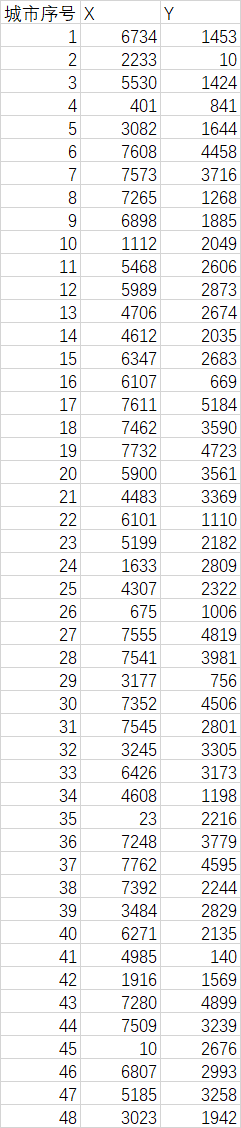项目导入：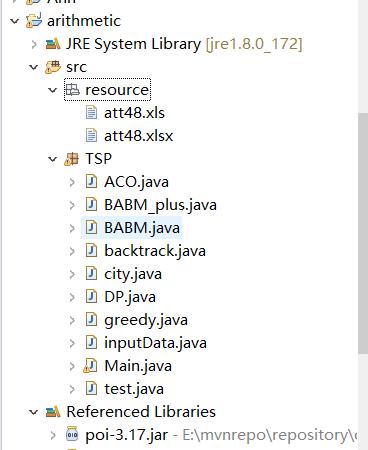3.实验主要源代码City.java//城市类，结构体package TSP;publicclass city {   privateintname;   privatedoubleX;   privatedoubleY;   public city(intname, doublex, doubley) {      super();      this.name = name-1;      X = x;      Y = y;   }   publicint getName() {      returnname;   }   publicvoid setName(intname) {      this.name = name;   }   publicdouble getX() {      returnX;   }   publicvoid setX(doublex) {      X = x;   }   publicdouble getY() {      returnY;   }   publicvoid setY(doubley) {      Y = y;   }   @Override   public String toString() {      return"city [name=" + name + ",X=" + X + ", Y=" + Y + "]";   }  } inputData.Java//导入数据类package TSP;import java.io.File;import java.io.FileInputStream;import java.io.FileNotFoundException;import java.io.IOException;import java.util.ArrayList;import java.util.List; import org.apache.poi.hssf.usermodel.HSSFRow;import org.apache.poi.hssf.usermodel.HSSFSheet;import org.apache.poi.hssf.usermodel.HSSFWorkbook; publicclass inputData {   @SuppressWarnings("resource")   publicstatic List<city> input_att48(File file){      List<city> cityList = new ArrayList<city>();      try {          HSSFWorkbook wookbook = new HSSFWorkbook(new FileInputStream(file));          HSSFSheet sheet = wookbook.getSheet("Sheet1");          introws = sheet.getPhysicalNumberOfRows();          for(inti=1; i<rows; i++){             HSSFRow row = sheet.getRow(i);             if(row!=null){                city cy = new city(i, row.getCell(1).getNumericCellValue(), row.getCell(2).getNumericCellValue());                cityList.add(cy);             }          }               } catch (FileNotFoundException e) {          System.out.println("File not fount!");      } catch (IOException e) {          System.out.println("IO exception!");      }      returncityList;   }} DP.Java//核心代码package TSP;import java.io.File;import java.util.List;import java.util.Scanner;public class DP {	//V集合表示已经旅行后的点的集合。	//n点经过集合V中所有点到达起始点的最短距离；1<<(n-1)代表一个二进制串，	//1代表该位置的城市在V集合例，反之不在。	static double INF = Double.MAX_VALUE;	static double[][] DT = null;	static double[][] DP = null;//	static int n = 0;	static void init(int n) {		File file = new File("E:\\Java\\arithmetic\\src\\resource\\att48.xls");		List<city> cityList = inputData.input_att48(file);		System.out.println("city [城市编号   城市X坐标    城市Y坐标]");		for(int i=0; i<n; i++) {			System.out.println(cityList.get(i).toString());		}		DT = new double[n][n];		DP = new double[n][1<<(n-1)];		for(int i=0; i<n; i++) {			for(int j=i; j<n; j++) {				if(i==j) DT[i][j] = 0;				else {					double dertX = cityList.get(i).getX()-cityList.get(j).getX();					double dertY = cityList.get(i).getY()-cityList.get(j).getY();					DT[i][j] = Math.sqrt(dertX*dertX + dertY*dertY);					DT[j][i] = DT[i][j];				}			}		}		//		for(int i=0; i<n; i++) {//			for(int j=0; j<n; j++) {//				System.out.print(DT[i][j]);//				System.out.print(" ");//			}//			System.out.println();//		}				//V集合为空的情况，初始化第i点直接回到起点s的距离		for(int i=1; i<n; i++) {			DP[i] = DT[i];		}	}		static void solve(int n) {		double minDt = INF;		double temp = 0;		for(int j=1; j<1<<(n-1); j++){//j的二进制表示V集合。	        for(int i=1; i<n; i++){//n个点减去起点还有n-1个点，遍历n-1个点选一个不在集合V中的点。	            if((1<<(i-1)&j)==0){//(1<<(i-1))&j==0表示i不在集合v中	                minDt = INF;	                for(int k=1; k<n; k++){	                    if(((1<<(k-1))&j)!=0){//(1<<(k-1))&j==1表示k在集合V中	                        temp = DT[i][k] + DP[k][j-(1<<(k-1))];	                        if(temp < minDt) minDt = temp;	                    }	                }	            }	            DP[i][j] = minDt;	        }	    }		minDt = INF;	    for(int k=1; k<n; k++){//1<<(9)=1000000000，((1<<(n-1))-1)=111111111111...	        temp = DT[k] + DP[k][((1<<(n-1))-1)-(1<<(k-1))];	        if(temp < minDt){	            minDt = temp;	        }	    }	    System.out.print("最短路径长：");	    System.out.println(minDt);	}		@SuppressWarnings("resource")	public static void main(String[] args) {		// TODO Auto-generated method stub		System.out.println("----------------动态规划解决TSP问题----------------");		Scanner in = new Scanner(System.in);		while(true) {			System.out.println();			System.out.println("请输入城市数：");			int n = in.nextInt();			if(n>24) {				System.out.println("城市数过多，请使用其他算法！");				return;			}			init(n);			solve(n);		}	}}输入输出：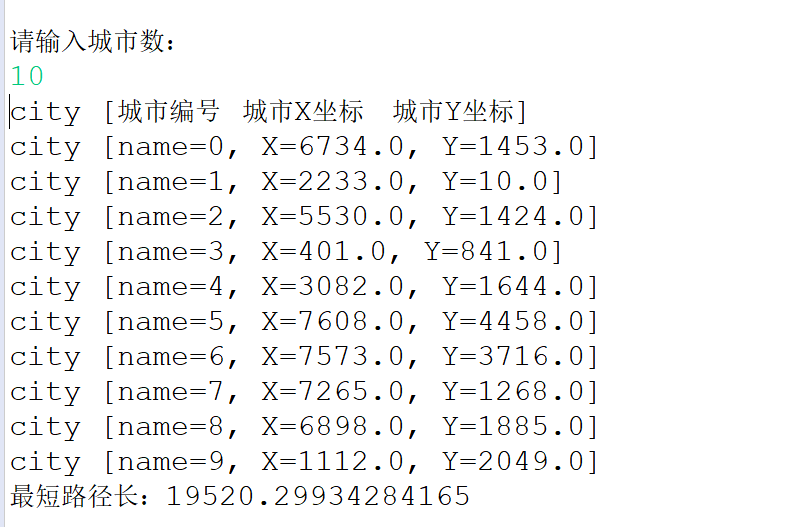展开全文• 动态规划算法根据的原理是，可以将原问题细分为规模更小的子问题，并且原问题的最优解中包含了子问题的最优解。也就是说，动态规划是一种将问题实例分解为更小的、相似的子问题，并存储子问题的解而避免计算重复的子...Python
• TSP 分别使用贪心算法、动态规划算法、分支限界法法解决以及蚁群算法解决n个城市的TSP问题。 输入格式： 城市编号 x y 0 100 230 1 678 349 . . .
• 动态规划算法解Travelling Salesman Problem（TSP问题旅行商问题 旅行商问题自动驾驶
• 能够使用C++语言编写出一个程序，这个程序能够实现一个功能，就是在网络 上找一条从 点出发，经过 各一次最后返回 的最短路线和最短路程。就是要求解决一个TSP问题
• 此ppt介绍了解决TSP（旅行商问题）的三种算法动态规划、蚁群算法、遗传算法
• 动态规划算法1.Levenshtein编辑距离DP算法2.0-1背包DP算法3.TSP问题DP算法 1.Levenshtein编辑距离DP算法 /** * @author: cuttle * @Date: 2020/10/21 19:42 * @Description: Levenshetein编辑距离DP算法 */ ...
• 动态规划法（少城市） 回溯法（中等规模城市数量） Sherwood概率算法改进版（随机第一个城市） 共8种算法（加粗的用于求解问题） 第一次发博客，如有错误，希望大佬们指正！ 问题及思路   1.问题概述 TSP问题是...c++
• 经典算法问题-TSP商旅问题（Traveling Salesman Problem），它是数学领域中著名问题之一。...代码包含遗传算法动态规划来求解这个问题，里面有完整源代码，并且有详细注释，还有两者的比较分析。
• 乍一看标题，大家是不是觉得“动态规划”这四个字组合在一起有点眼熟？似乎哪会儿学过来着……但是吧，细细一琢磨，又忘了它具体是什么、怎么用、用来...什么是TSP动态规划 简单来说，Travelling Salesman Proble...
• 针对蚁群算法搜索空间大、收敛速度慢、容易陷入局部最优等缺陷，提出一种基于动态凸包引导的偏优规划蚁群算法。改进后的算法动态控制蚂蚁的待选城市范围，有助于在跳出局部最优并向全局最优逼近的基础上减少蚂蚁搜索...二维凸包
• 双调欧几里得旅行商问题是一个经典动态规划问题。《算法导论（第二版）》思考题15-1和北京大学OJ2677都出现了这个题目。 旅行商问题描述：平面上n个点，确定一条连接各点的最短闭合旅程。这个解的一般形式为NP的...
• 问题描述不展开了，感兴趣可以自己搜一下。csdn上这篇文章介绍的很详细，可以看一下 ，http://blog.csdn.net/q345852047/article/details/6626684 感谢作者辛勤码字，我就偷懒啦～1.贪心"""Functions:find_path:Data...
• 乍一看标题，大家是不是觉得“动态规划”这四个字组合在一起有点眼熟？似乎哪会儿学过来着……但是吧，细细一琢磨，又忘了它具体是什么、怎么用、用来...什么是TSP动态规划 简单来说，Travelling Salesman Proble...
• 2、使用动态规划法编程，求解0/1背包问题和TSP问题TSP问题 一、实验内容： TSP问题是指旅行家要旅行n个城市，要求每个城市经历且仅经历一次然后回到出发城市，并要求所走的路程最短。 对于图G=(V,E),假设从顶点i...
• 2016-03-17 20:35:36利用动态规划方法是可以精确求解旅行推销商问题(Traveling Salesman Problem)的, 虽然这种方法只适用于求解小规模的问题. 这个算法我一直没有弄清楚, 最近有个问题需要使用类似的算法来解决, ...
• php大佬们，请教一个问题 最近在看动态规划解决tsp算法问题 我百度不到php代码 所以根据别的语言翻译出来一个php版的 但是运行会出现问题 文章链接： ...depth_1-utm_source=php
• TSP问题 TSP问题是什么？ TSP问题是指旅行家要旅行n个城市，要求各个城市经历且仅经历一次然后回到出发城市，并要求所走的路线图最短。 题目 带权图的代价矩阵 无穷 3 6 7 5 无穷 2 3 6 4 无穷 2 6 7 5 ...
• ## 动态规划法求解TSP问题 C++

万次阅读 多人点赞 2018-07-01 14:06:16
“鸡汤惠”帮“鸭汤莹”看代码，于是翻出了自己写的动态规划法求解TSP问题，于是整理了一下。（算法思想在知识点整理的部分，这里是具体实现的代码） 问题描述：  TSP问题是指旅行家要旅行n个城市，要求各个城市...
• 算法设计中的TSP问题的蛮力法、动态规划法、贪心法及回溯法。
• TSP问题描述： 　旅行商问题，即TSP问题（Travelling Salesman Problem）又译为旅行推销员问题、货郎担问题，是数学领域中著名问题之一。假设有一个旅行商人要拜访n个城市，他必须选择所要走的路径，路径的限制是......